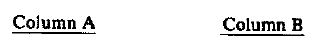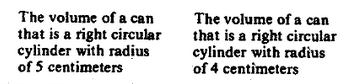# Quantitative Comparison Example Problem

The Quantitative Comparison question type on the GRE can be very challenging. Essentially, you are given some information (usually in the form of a sentence, equation, or picture) and then two quantities. You are then asked to determine:

A if the quantity in Column A is greater;

B if the quantity in Column B is greater;

C if the two quantities are equal;

D is the relationship cannot be determined from the information given.

Like most questions on the GRE Quantitative section, the concepts involved in solving this question type are not of themselves very advanced, you just have to be careful in their application. In fact, sometimes there is little or no need at all to do any “real” math; let’s take a look at the following GRE Quantitative Comparison Example Problem:With geometry problems, it always helps to start with a formula. In this case, the problem is involving the volume of a cylinder. The formula for this is pi times the radius squared times the height.

Volume of a cylinder = πr2h

Therefore, to find the volume of a cylinder you must find both the radius of the cylinder and the height.

In this problem, two cylinders are given. Both cylinders have a different radius, and so it might be assumed that they must have a different volume. However, no information about the height is given. It is possible that the cylinder with the smaller radius has a larger height and might be the larger cylinder. Since there is no way of knowing how these cylinders compare to each other, the answer is D.

Test Masters offers the most comprehensive and successful GRE course available; every Test Masters GRE course, whether it is online or in-class, comes with a 10 point Score Increase Guarantee.

This entry was posted in Example Problems, Revised GRE and tagged , , , . Bookmark the permalink.